Get inspired by the success stories of our students in IIT JAM MS, ISI  MStat, CMI MSc DS.  Learn More

## ISI MStat 2020 PSB Problem 6

This post discuses the problem 6 of the ISI MStat 2020 PSB Entrance Exam.

Suppose individuals are classified into three categories C1,C2$C_{1}, C_{2}$ and C3$C_{3}$.

Let p2,(1p)2$p^{2},(1-p)^{2}$ and 2p(1p)$2 p(1-p)$ be the respective population proportions, where p∈(0,1)$p \in (0,1)$. A random sample of N$N$ individuals is selected from the population and the category of each selected individual recorded.

For i=1,2,3$i=1,2,3$, let Xi$X_{i}$ denote the number of individuals in the sample belonging to category Ci$C_{i}$. Define U=X1+X32$U=X_{1}+\frac{X_{3}}{2}$.

• Is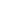sufficient for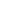? Justify your answer.
• Show that the mean squared error of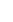is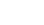.

## Hints, Solution, and More

• Prove that the joint distribution of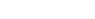follows Multinomial Distribution.
• Write the Likelihood of the data.
• Use Neymann Factorization to prove the sufficiency of.
• Show thatis unbiased.
• Show that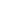follows Binomial Distribution.

Do subscribe to our channel to get instant notification of Live Session, so that you can join us live in the master classes!

Learn. Enjoy. Practice. Repeat.

• Prove thatis the UMVUE of.
• Find the minimal sufficient and complete statistic of.
• For other Food for Thought, refer to the youtube video for full solution.

### Knowledge Partner